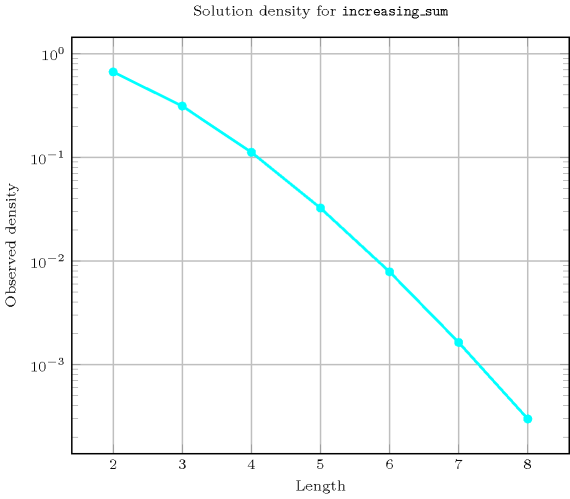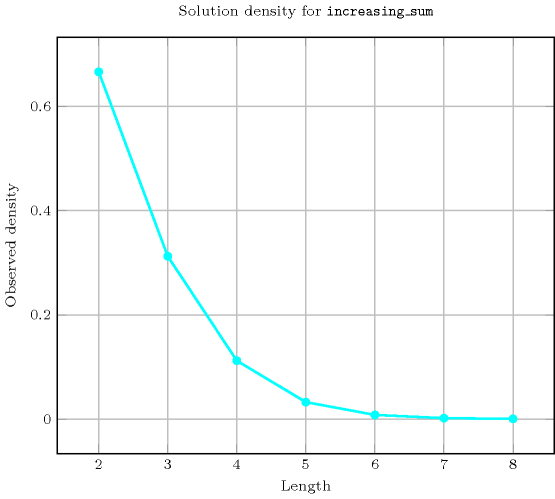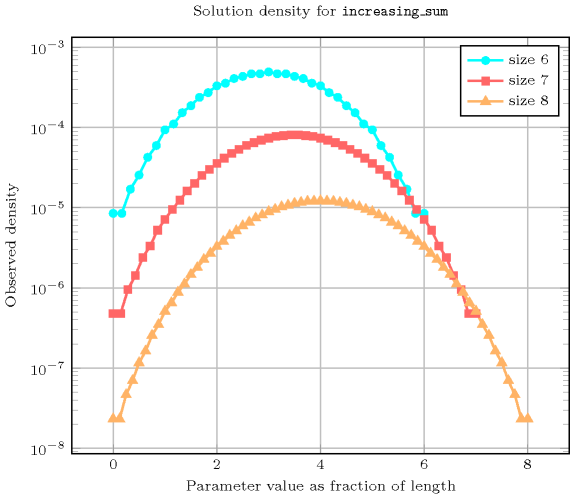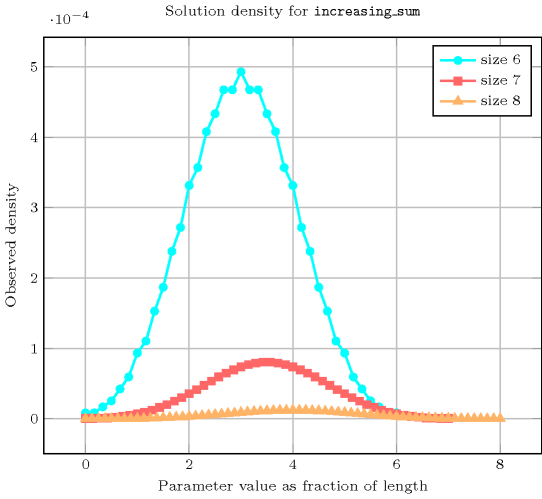## 5.190. increasing_sum

Origin
Constraint

$\mathrm{𝚒𝚗𝚌𝚛𝚎𝚊𝚜𝚒𝚗𝚐}_\mathrm{𝚜𝚞𝚖}\left(\mathrm{𝚅𝙰𝚁𝙸𝙰𝙱𝙻𝙴𝚂},𝚂\right)$

Synonyms

$\mathrm{𝚒𝚗𝚌𝚛𝚎𝚊𝚜𝚒𝚗𝚐}_\mathrm{𝚜𝚞𝚖}_\mathrm{𝚌𝚝𝚛}$, $\mathrm{𝚒𝚗𝚌𝚛𝚎𝚊𝚜𝚒𝚗𝚐}_\mathrm{𝚜𝚞𝚖}_\mathrm{𝚎𝚚}$.

Arguments
 $\mathrm{𝚅𝙰𝚁𝙸𝙰𝙱𝙻𝙴𝚂}$ $\mathrm{𝚌𝚘𝚕𝚕𝚎𝚌𝚝𝚒𝚘𝚗}\left(\mathrm{𝚟𝚊𝚛}-\mathrm{𝚍𝚟𝚊𝚛}\right)$ $𝚂$ $\mathrm{𝚍𝚟𝚊𝚛}$
Restrictions
 $\mathrm{𝚛𝚎𝚚𝚞𝚒𝚛𝚎𝚍}$$\left(\mathrm{𝚅𝙰𝚁𝙸𝙰𝙱𝙻𝙴𝚂},\mathrm{𝚟𝚊𝚛}\right)$ $\mathrm{𝚒𝚗𝚌𝚛𝚎𝚊𝚜𝚒𝚗𝚐}$$\left(\mathrm{𝚅𝙰𝚁𝙸𝙰𝙱𝙻𝙴𝚂}\right)$
Purpose

The variables of the collection $\mathrm{𝚅𝙰𝚁𝙸𝙰𝙱𝙻𝙴𝚂}$ are increasing. In addition, $𝚂$ is the sum of the variables of the collection $\mathrm{𝚅𝙰𝚁𝙸𝙰𝙱𝙻𝙴𝚂}$.

Example
$\left(〈3,3,6,8〉,20\right)$

The $\mathrm{𝚒𝚗𝚌𝚛𝚎𝚊𝚜𝚒𝚗𝚐}_\mathrm{𝚜𝚞𝚖}$ constraint holds since:

• The values of the collection $〈3,3,6,8〉$ are sorted in increasing order.

• $𝚂=20$ is set to the sum $〈3+3+6+8〉$.

Typical
 $|\mathrm{𝚅𝙰𝚁𝙸𝙰𝙱𝙻𝙴𝚂}|>1$ $\mathrm{𝚛𝚊𝚗𝚐𝚎}$$\left(\mathrm{𝚅𝙰𝚁𝙸𝙰𝙱𝙻𝙴𝚂}.\mathrm{𝚟𝚊𝚛}\right)>1$
Arg. properties

Functional dependency: $𝚂$ determined by $\mathrm{𝚅𝙰𝚁𝙸𝙰𝙱𝙻𝙴𝚂}$.

Usage

The $\mathrm{𝚒𝚗𝚌𝚛𝚎𝚊𝚜𝚒𝚗𝚐}_\mathrm{𝚜𝚞𝚖}$ constraint can be used for breaking some symmetries in bin packing problems. Given a set of $n$ bins with the same maximum capacity, and a set of items each of them with a specific height, the problem is to pack all items in the bins. To break symmetry we order bins by increasing use. This is done by introducing a variable ${x}_{i}$ $\left(0\le i for each bin $i$ giving its use, i.e., the sum of items heights assigned to bin $i$, and by posting the following $\mathrm{𝚒𝚗𝚌𝚛𝚎𝚊𝚜𝚒𝚗𝚐}_\mathrm{𝚜𝚞𝚖}$$\left(〈{x}_{0},{x}_{1},\cdots ,{x}_{n-1}〉,s\right)$ where $s$ denotes the sum of the heights of all the items to pack.

Algorithm

A linear time filtering algorithm achieving bound-consistency for the $\mathrm{𝚒𝚗𝚌𝚛𝚎𝚊𝚜𝚒𝚗𝚐}_\mathrm{𝚜𝚞𝚖}$ constraint is described in [PetitReginBeldiceanu11]. This algorithm was motivated by the fact that achieving bound-consistency on the inequality constraints and on the sum constraint independently hinders propagation, as illustrated by the following small example, where the maximum value of ${x}_{1}$ is not reduced to 2: ${x}_{1}\in \left[1,3\right]$, ${x}_{2}\in \left[2,5\right]$, $s\in \left[5,6\right]$, ${x}_{1}<{x}_{2}$, ${x}_{1}+{x}_{2}=s$.

Given an $\mathrm{𝚒𝚗𝚌𝚛𝚎𝚊𝚜𝚒𝚗𝚐}_\mathrm{𝚜𝚞𝚖}$$\left(〈{x}_{0},{x}_{1},\cdots ,{x}_{n-1}〉,s\right)$ constraint, the bound-consistency algorithm consists of three phases:

1. A normalisation phase adjusts the minimum and maximum value of variables ${x}_{0},{x}_{1},\cdots ,{x}_{n-1}$ with respect to the chain of inequalities ${x}_{0}\le {x}_{1}\le \cdots \le {x}_{n-1}$. A forward phase adjusts the minimum value of ${x}_{1},{x}_{2},\cdots ,{x}_{n-1}$ (i.e., $\underline{{x}_{i+1}}\ge \underline{{x}_{i}}$), while a backward phase adjusts the maximum value of ${x}_{n-2},{x}_{n-1},\cdots ,{x}_{0}$ (i.e., $\overline{{x}_{i-1}}\le \overline{{x}_{i}}$).

2. A phase restricts the minimum and maximum value of the sum variable $s$ with respect to the chain of inequalities ${x}_{0}\le {x}_{1}\le \cdots \le {x}_{n-1}$ (i.e., $\underline{s}\ge {\sum }_{0\le i and $\overline{s}\le {\sum }_{0\le i).

3. A final phase reduces the minimum and maximum value of variables ${x}_{0},{x}_{1},\cdots ,{x}_{n-1}$ both from the bounds of $s$ and from the chain of inequalities. Without loss of generality we now focus on the pruning of the maximum value of variables ${x}_{0},{x}_{1},\cdots ,{x}_{n-1}$. For this purpose we first need to introduce the notion of last intersecting index of a variable ${x}_{i}$, denoted by ${\mathrm{𝑙𝑎𝑠𝑡}}_{i}$. This corresponds to the greatest index in $\left[i+1,n-1\right]$ such that $\overline{{x}_{i}}>\underline{{x}_{{\mathrm{𝑙𝑎𝑠𝑡}}_{i}}}$, or $i$ if no such integer exists. Then the increase of the minimum value of $s$ when ${x}_{i}$ is equal to $\overline{{x}_{i}}$ is equal to ${\sum }_{k\in \left[i,{\mathrm{𝑙𝑎𝑠𝑡}}_{i}\right]}\left(\overline{{x}_{i}}-\underline{{x}_{k}}\right)$. When this increase exceeds the available margin, i.e. $\overline{s}-{\sum }_{0\le i, we update the maximum value of ${x}_{i}$.

We illustrate a part of the final phase on the following example $\mathrm{𝚒𝚗𝚌𝚛𝚎𝚊𝚜𝚒𝚗𝚐}_\mathrm{𝚜𝚞𝚖}$$\left(〈{x}_{0},{x}_{1},{x}_{2},{x}_{3},{x}_{4},{x}_{5}〉,s\right)$, where ${x}_{0}\in \left[2,6\right]$, ${x}_{1}\in \left[4,7\right]$, ${x}_{2}\in \left[4,7\right]$, ${x}_{3}\in \left[5,7\right]$, ${x}_{4}\in \left[6,9\right]$, ${x}_{5}\in \left[7,9\right]$ and $s\in \left[28,29\right]$. Observe that the domains are consistent with the first two phases of the algorithm, since,

1. the minimum (and maximum) values of variables ${x}_{0},{x}_{1},{x}_{2},{x}_{3},{x}_{4},{x}_{5}$ are increasing,

2. the sum of the minimum of the variables ${x}_{0},{x}_{1},{x}_{2},{x}_{3},{x}_{4},{x}_{5}$, i.e., 28 is less than or equal to the maximum value of $s$,

3. the sum of the maximum of the variables ${x}_{0},{x}_{1},{x}_{2},{x}_{3},{x}_{4},{x}_{5}$, i.e., 45 is greater than or equal to the minimum value of $s$.

Now, assume we want to know the increase of the minimum value of $s$ when ${x}_{0}$ is set to its maximum value 6. First we compute the last intersecting index of variable ${x}_{0}$. Since ${x}_{4}$ is the last variable for which the minimum value is less than or equal to maximum value of ${x}_{0}$ we have ${\mathrm{𝑙𝑎𝑠𝑡}}_{0}=4$. The increase is equal to ${\sum }_{k\in \left[0,4\right]}\left(\overline{{x}_{0}}-\underline{{x}_{k}}\right)=\left(6-2\right)+\left(6-4\right)+\left(6-4\right)+\left(6-5\right)+\left(6-6\right)=9$. Since it exceeds the margin $29-\left(2+4+4+5+6+7\right)=1$ we have to reduce the maximum value of ${x}_{0}$. How to do this incrementally is described in [PetitReginBeldiceanu11].

Counting
 Length ($n$) 2 3 4 5 6 7 8 Solutions 6 20 70 252 924 3432 12870

Number of solutions for $\mathrm{𝚒𝚗𝚌𝚛𝚎𝚊𝚜𝚒𝚗𝚐}_\mathrm{𝚜𝚞𝚖}$: domains $0..n$Length ($n$)2345678
Total62070252924343212870
 Parameter value

01111111
11111111
22222222
31333333
41355555
5-357777
6-379111111
7-2711131515
8-1814182022
9-1716222628
10--718283438
11--519324248
12--520395363
13--320426377
14--219487597
15--1185187116
16--11655100141
17---1455112164
18---1158125194
19---955136221
20---755146255
21---551155284
22---348162319
23---242166348
24---139169383
25---132169409
26----28166440
27----22162461
28----18155486
29----13146499
30----11136515
31----7125519
32----5112526
33----3100519
34----287515
35----175499
36----163486
37-----53461
38-----42440
39-----34409
40-----26383
41-----20348
42-----15319
43-----11284
44-----7255
45-----5221
46-----3194
47-----2164
48-----1141
49-----1116
50------97
51------77
52------63
53------48
54------38
55------28
56------22
57------15
58------11
59------7
60------5
61------3
62------2
63------1
64------1

Solution count for $\mathrm{𝚒𝚗𝚌𝚛𝚎𝚊𝚜𝚒𝚗𝚐}_\mathrm{𝚜𝚞𝚖}$: domains $0..n$Keywords
Cond. implications

$•$ $\mathrm{𝚒𝚗𝚌𝚛𝚎𝚊𝚜𝚒𝚗𝚐}_\mathrm{𝚜𝚞𝚖}\left(\mathrm{𝚅𝙰𝚁𝙸𝙰𝙱𝙻𝙴𝚂},𝚂\right)$

with  $\mathrm{𝚖𝚒𝚗𝚟𝚊𝚕}$$\left(\mathrm{𝚅𝙰𝚁𝙸𝙰𝙱𝙻𝙴𝚂}.\mathrm{𝚟𝚊𝚛}\right)>0$

implies $\mathrm{𝚊𝚝𝚖𝚘𝚜𝚝}_\mathrm{𝚗𝚟𝚊𝚕𝚞𝚎}$$\left(𝚂,\mathrm{𝚅𝙰𝚁𝙸𝙰𝙱𝙻𝙴𝚂}\right)$.

$•$ $\mathrm{𝚒𝚗𝚌𝚛𝚎𝚊𝚜𝚒𝚗𝚐}_\mathrm{𝚜𝚞𝚖}\left(\mathrm{𝚅𝙰𝚁𝙸𝙰𝙱𝙻𝙴𝚂},𝚂\right)$

with  $\mathrm{𝚖𝚒𝚗𝚟𝚊𝚕}$$\left(\mathrm{𝚅𝙰𝚁𝙸𝙰𝙱𝙻𝙴𝚂}.\mathrm{𝚟𝚊𝚛}\right)>0$

implies $\mathrm{𝚜𝚞𝚖}_\mathrm{𝚘𝚏}_\mathrm{𝚒𝚗𝚌𝚛𝚎𝚖𝚎𝚗𝚝𝚜}$$\left(\mathrm{𝚅𝙰𝚁𝙸𝙰𝙱𝙻𝙴𝚂},\mathrm{𝙻𝙸𝙼𝙸𝚃}\right)$.